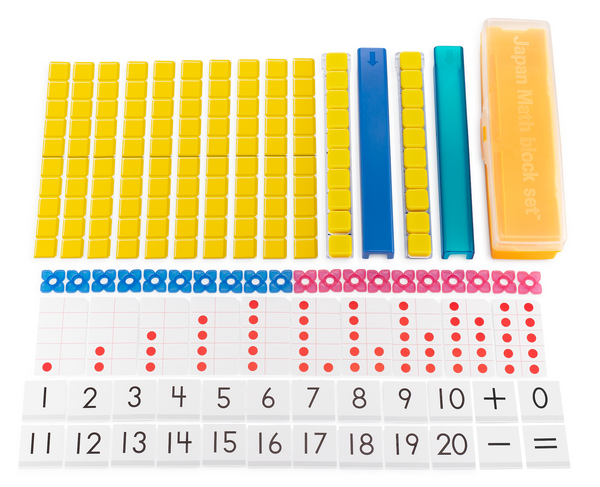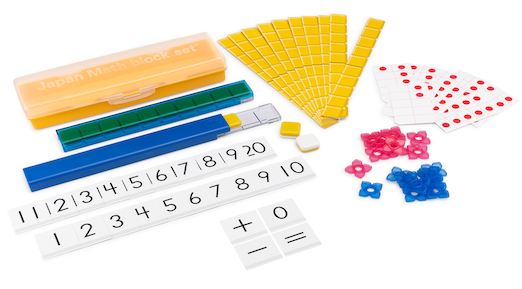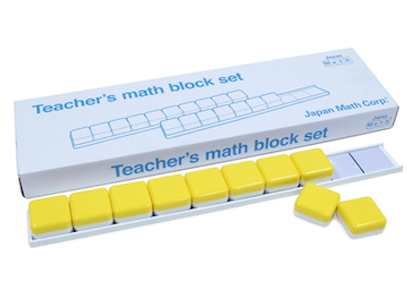# Math Manipulatives – Using Japan Math Block Sets

### What are manipulatives?

Math manipulatives are essential tools for young students to learn mathematics. In the early stages of learning, pictures do not enhance the physical component of learning like our math manipulatives. Children grasp the concepts of numbers by using their hands and experiencing the increase or decrease of objects. Japan Math Block Set features distinctive items that maximize the effect of any math lessons.

The idea of making “a group of 10” becomes fundamental for understanding the concept of base-10 numbers. Therefore, this idea will become a foundation when learning to calculate larger numbers such as 20 and 30. The idea of making “a group of 10’ is the basis for learning addition and subtraction of larger numbers.

Japan Math Block Sets are helpful in deepening students’ understanding of addition where the making-10 strategy is used. They are also effective as a tool to communicate their ideas and their understanding to others. A student who has understood the material can help a student who is struggling to understand by verbal explanation accompanied by block manipulation. There are many cases in which verbal communication is made easier when accompanied by the manipulation of objects.

### What is included in the Japan Math Block Sets?

As effective tools for helping young students acquire number sense and knowledge about number structure, “Counters,” “Ten Frame Cards,” and “Number Cards’ are provided in addition to the block manipulatives in the set. These items allow students to engage in activities such as the “Card-turning Game” and “Number-guessing Game,” and increase their interest and motivation for learning. At the same time, learning through hands-on experience and enjoyment will make it easier for students to understand subsequent mathematical concepts.Item Features
(1) Math Blocks (10 in clear case) Students compare numbers and compose and decompose numbers while naturally building their awareness of a “set of 10”.
(2) Math Blocks (10 in opaque case) Once students understand composition and decomposition using the clear case, they can practice making 10's using the opaque case.
(3) Math Block Picture Cards (10) For counting numbers from 10 to 100 as a substitute for math blocks.
(4) Counters (10 red and 10 blue) For counting objects and playing various number-related games.
(5) Red Dot Cards (10) Cards, with dots ranging from 1 to 10, work as a bridge to connect the number of concrete objects to numerical numbers.
(6) Number Cards (20) Cards, with numerals ranging from 1 to 20, teach how numbers correspond to numerals, which are abstract symbols of numbers.

### Product LineupJapan Math Block Set (Kindergarten - Grade 1)

A set of essential tools for young children designed to help them understand the concept of numbers.Teacher's Block Set (Kindergarten - Grade 1)

Big magnetic math blocks to be used on whiteboards for explaining the operation of blocks to students.